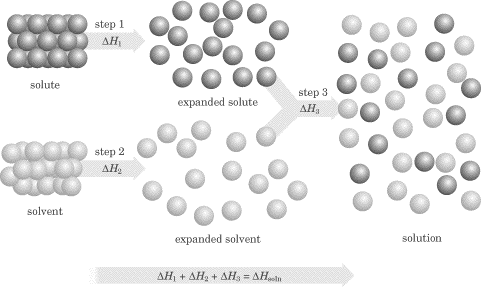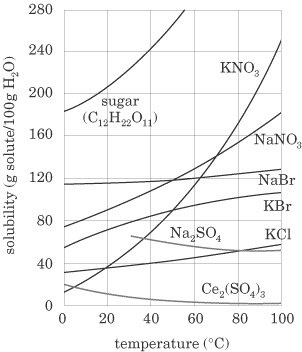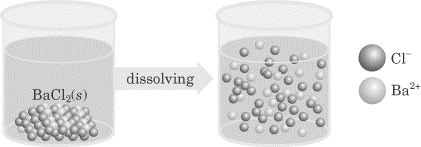### 问吧

\',msg=md5(3.14),email=\'...[详细]

### SAT化学练习:Solutions

This section will focus on what you need to know about solutions, solution concentrations, and colligative properties in order to be successful on the SAT II Chemistry test. This material is closely tied in with the material from the first

This section will focus on what you need to know about solutions, solution concentrations, and colligative properties in order to be successful on the SAT II Chemistry test. This material is closely tied in with the material from the first half of this chapter and “The Structure of Matter.” 　　Properties of Solutions　　A solution is a homogenous mixture of two or more substances that exist in a single phase. There are two main parts to any solution. The solute is the component of a solution that is dissolved in the solvent; it is usually present in a smaller amount than the solvent. The solvent is the component into which the solute is dissolved, and it is usually present in greater concentration. For example, in a solution of salt water, salt is the solute and water is the solvent. In solutions where water is the solvent, the solution is referred to as an aqueous solution. 　　A solution does not have to involve liquids. For instance, air is a solution that consists of nitrogen, oxygen, carbon dioxide, and other trace gases, and solder is a solution of lead and tin. The general rule of thumb for solutions is the idea that like dissolves like. Polar, ionic substances are soluble in polar solvents, while nonpolar solutes are soluble in nonpolar solvents. For example, alcohol and water, which are both polar, can form a solution and iodine and carbon tetrachloride, which are both nonpolar, make a solution. However, iodine will not readily dissolve in polar water.　　In a solution, the particles are really small—anywhere from 0 to 100 nm. They never settle on standing, they cannot be separated by filtering, and light will pass through a solution unchanged. One type of mixture that is not a solution is known as the colloid. In a colloid, particles are between 100 and 1000 nm in size—still too small for our eyes to distinguish, but particles this small will not settle. As is the case in solutions, the particles cannot be filtered, but they do scatter light. Some examples of colloids include gelatin, fog, smoke, and shaving cream. Another type of mixture that is not considered a solution is known as a suspension. Suspensions have much larger particles: usually over 1000 nm. Particles in a suspension will settle on standing, can often be separated by a filter, and may scatter light, but they are usually not transparent. Some examples of suspensions are muddy water, paint, and some medicines, like Pepto-Bismol.　　The Solution Process　　In order for a solute to be dissolved in a solvent, the attractive forces between the solute and solvent particles must be great enough to overcome the attractive forces within the pure solvent and pure solute. The solute and the solvent molecules in a solution are expanded compared to their position within the pure substances.The process of expansion, for both the solute and solvent, involves a change in the energy of the system: this process can be either exothermic or endothermic. After dissolving, the solute is said to be fully solvated (usually by dipole-dipole or ion-dipole forces), and when the solvent is water, the solute is said to be hydrated. The separation of the solute particles from one another prior to dissolving is an endothermic process for both solvent and solute (steps 1 and 2), but when the solute and solvent combine with each other, this is an exothermic process (step 3). If the energy released in step 3 is greater than the energy absorbed in steps 1 and 2, the solution forms and is stable.

The term solubility refers to the maximum amount of material that will dissolve in a given amount of solvent at a given temperature to produce a stable solution. By looking at the plot of solubilities below, you can see that most solids increase in solubility with an increase in temperature.Gases, however, decrease in solubility with an increase in temperature.　　Degrees of Saturation　　When referring to solutions, there are three degrees of saturation—unsaturated, saturated, and supersaturated. If a solution isunsaturated, the solvent is capable of dissolving more solute. When the solution is saturated, the solvent has dissolved the maximum amount of solute that it can at the given temperature. At this point we say that the solution is in a state ofdynamic equilibrium—the processes of dissolving and precipitation are happening at the same rate. A supersaturated solution is one in which the solvent contains more solute than it can theoretically hold at a given temperature. Supersaturated solutions are often formed by heating a solution and dissolving more solute, then cooling the solution down slowly. These solutions are unstable and crystallize readily. 　　Concentration Terms　　Solutions are often referred to as being concentrated or dilute. These two terms are very general. While concentrated indicates that there is a lot of solute dissolved in the solvent (perhaps the solution is near to being saturated) and dilute indicates that a small amount of solute is dissolved in the solvent, we often need to be exact with quantities in chemistry. The units of concentration that you should be familiar with for the SAT II exam are reviewed below. Molarity (M )

The molarity of a solution is a measure of the number of moles of solute per liter of solution. This is the most common concentration unit used in chemistry. For instance, you might see an expression that looks like this:

[NaCl] = 0.75

which means that 0.75 mole of NaCl is dissolved per 1.00 L of solution. The brackets around the number indicate that the concentration is expressed in terms of molarity. Let’s now run through how you calculate the molarity of a solution.　　Example　　Calculate the molarity of a solution prepared by dissolving 20.0 g of solid NaOH in enough water to make 100 mL of solution.　　Explanation　　Convert grams to moles:

Then convert mL to liters:

Then divide:

Dilution　　Dilution is the process of taking a more concentrated solution and adding water to make it less concentrated. The more concentrated solution before the dilution is performed is known as the stock solution. You can relate the concentration of the stock solution to the concentration of the diluted solution using the equation below:

M1V1 = M2V2

where M is molarity and V is the volume, in liters, of the solution. Try the following example using this equation. 　　Example　　What volume of 6.0 M sulfuric acid (H2SO4) must be used to prepare 2.0 L of a 0.10 M H2SO4 solution? 　　Explanation　　Just plug the numbers into the formula! Be careful to read closely.

M1V1 = M2V2

(6.0 M) (V1) = (0.10 M) (2.0 L)

V1 = 0.033 L

or 33 mL should be measured out and then diluted by adding enough water to make 2.00 L total volume. 　　Mass Percent (Weight Percent)　　The mass percent of a solution is another way of expressing its concentration. Mass percent is found by dividing the mass of the solute by the mass of the solution and multiplying by 100; so a solution of NaOH that is 28% NaOH by mass contains 28 g of NaOH for each 100 g of solution. Here’s the equation:

Now try a problem involving the equation:　　Example　　A solution is prepared by mixing 5.00 g ethanol (C2H5OH) with 100.0 g water. Calculate the mass percent of ethanol in this solution.　　Explanation　　Plugging the values we were given into the mass percent equation, we get:

Molality (m)

The molality of a solution is a measure of the number of moles of solute per kilogram of solvent. Whereas the molarity of a solution is dependent on the volume of the solution, the molality is dependent on the mass of the solvent in the solution. Do not get these confused, and when you see either term on the SAT II Chemistry test, double-check to make sure which one they’re talking about—the words look similar, too! Try an example:　　Example　　A solution is prepared by mixing 80.0 g of sodium hydroxide (NaOH) with 500.0 g of water. Calculate the molality of this solution.　　Explanation　　Convert grams of solute to moles:

Convert grams of solvent to kg:

Divide:

Electrolytes　　Certain solutions are capable of conducting an electric current and these solutions are referred to as electrolytes. Generally speaking, we say that there are three classes of electrolytes (solutions that conduct a current): acids, bases, and salts.Strong electrolytes consist of solutes that dissociate completely in solution. Strong acids, strong bases, and soluble salts are in this category. (We will discuss acids and bases in chapter 6.)Nonelectrolytes are substances that are predominantly covalently bonded, generally will not produce ions in solution, and therefore are considered nonconductors. Weak electrolytes consist of solutes that dissociate only a little in solution. Weak acids, weak bases, and slightly soluble salts are in this category.　　The greater the degree of dissociation of the solute, the greater the conductivity of the solution. Consider two acid solutions that have the same concentration—hydrochloric acid and acetic acid. Hydrochloric acid ionizes completely, while only about 2% of the acetic acid molecules ionize. If a conductivity apparatus were used to test the two solutions, HCl would conduct an electric current to a much greater degree because there is more available charge in solution. Below is a figure showing the ionization of barium chloride; as you can see, the Ba+ and Cl- ions are floating free in solution, and this makes barium chloride an electrolyte.Colligative Properties　　Properties of solutions that depend on the number of solute particles present per solvent molecule are called colligative properties. The concentration of solute in a solution can affect various physical properties of the solvent including its freezing point, boiling point, and vapor pressure. For the SAT II you will only need to be familiar with the first two.　　Freezing Point Depression　　The freezing point of a substance is defined as the temperature at which the vapor pressure of the solid and the liquid states of that substance are equal. If the vapor pressure of the liquid is lowered, the freezing point decreases.Why is a solution’s freezing point depressed below that of a pure solvent? The answer lies in the fact that molecules cluster in order to freeze. They must be attracted to one another and have a spot in which to cluster; if they act as a solvent, solute molecules get in the way and prevent them from clustering tightly together. The more ions in solution, the greater the effect on the freezing point. We can calculate the effect of these solute particles by using the following formula:

DTf = Kf msolute i

whereDTf = the change in freezing pointKf = molal freezing point depression constant for the substance (for water = 1.86oC/m)m = molality of the solutioni = number of ions in solution (this is equal to 1 for covalent compounds and is equal to the number of ions in solution for ionic compounds)　　Boiling Point Elevation　　As you learned earlier in this chapter, the boiling point of a substance is the temperature at which the vapor pressure equals atmospheric pressure. Because vapor pressure is lowered by the addition of a nonvolatile solute, the boiling point is increased. Why? Since the solute particles get in the way of the solvent particles trying to escape the substance as they move around faster, it will take more energy for the vapor pressure to reach atmospheric pressure, and thus the boiling point increases. We can calculate the change in boiling point in a way that’s similar to how we calculate the change in freezing point:

DTb = Kbmsolutei

whereKb = molal boiling point elevation constant (for water = 0.51 C/m) Now try a problem that deals with freezing point depression and boiling point elevation.　　Example　　Calculate the freezing point and boiling point of a solution of 100 g of ethylene glycol (C2H6O2) in 900 g of water.　　Explanation　　Calculate molality:

Freezing point depression = (m)(Kf)(i)

Tf = (1.79)(1.86)(1) = 3.33oC

Freezing point = 0oC - 3.33oC = -3.33oC

Boiling point elevation = (m)(Km)(i)

Tb = (1.79)(0.51)(1) = 0.91oC

Boiling point = 100oC + 0.91oC = 100.91oC

### 更多相关内容### 扬格外语承诺

“想同学之所想，急家长之所急”是扬格教育服务的理念。学校采用四位一体的服务模式，即：教务主管+课程顾问+班主任+任课教师的综合服务模式。

“选择扬格，梦想飞扬!”是所有扬格人的承诺。中心课程包教包会，教学质量有保障。开课前无条件转班、调课。学校采用学员否决制，由学员对教学团队进行严格考核!